## How much rail do we need?

Every solar project requires the use of framing or rail to support the panels so structural integrity is maintained, the manufacturer’s warranty is abided by and the system is safe. How many rail lengths are needed; how many mid and end clamps; and how many  rail joiners are questions every designer and operations manager asks.

This blog will discuss a basic approach using a spreadsheet to calculate what is actually needed. The program referenced is Excel for MAC but any spreadsheet software can be used.

The format follows an input and output approach and as can be seen in the image above, there is a Solar panel Framing Inputs table and a Solar panel Framing Outputs table.

The first input is the panel brand, followed by the model, length of the panel, the width and the number of panels. These inputs are referenced in formulas that derive the outputs. The questions Is this one string yes or no can determine the number of DC isolators used and the question asking Is row separate determines the number of end clamps used.

With solar panel framing the main components are the frame or rail, top and bottom, mid clamps, end clamps and frame rail joiners.

## Spacings and buffers are important!

As we progress down the rows we come to questions concerning the spacings required for the mid and end clamps and a number, in millimetres, is used. This information is derived from the manufacturer’s data sheet but the buffer amount is not. The buffer amount is “insurance” because of the issue of tolerances and mistakes. For example, consider 19 x panels on a separate row with 18 x spaces for the mid clamps - depending on how tightly the panels are pushed against the mid clamps there will be creep. The buffer amount takes this into consideration.

## Length of rail used and DC isolator allowance

Depending on the manufacturer and the model of rail used there are different lengths available. Usually on a project one length is selected (as per the designer’s suggestion). Now the Top rail additional length for DC relates to the amount of rail used to house the DC isolator and shroud. In the example, I have allowed 300mm but this is up to the designer.

## Solar panel Framing Inputs summary

1. Input into the cells that have, in this case, a yellow background
2. Make sure that the information is correct (especially the numbers)
3. Always check the manufacturer’s data sheets

## Now for the outputs

In this very basic example, the outputs consist of the total length of rail required for the 19 x panels.

See below.

## What are the formulas involved?

Now, to determine the top rail length the formula has to collect information from the input table. I have shown exactly what is required by using precedents; the blue arrows show that the formula in G3 uses almost all the inputs.

## The formula in detail

I have used an if statement that asks a question and based on the answer, produces a particular result.

So if the answer to the question is the system Portrait or Landscape, P or L, is P, which it is, the formula takes the panel width figure of 1000 and multiplies by the number of panels, which is 19, so have 19,000 mm.

Then 1 is subtracted from 19 to ascertain the number of gaps between the panels then multiplied by the number in mm in C11 ( the mid clamp gap). To this we add the end clamp amounts in C12 and multiply by 2 because there are two ends.

But it’s not over yet as we have to add the buffer amount in C13 and, like the end clamps, we multiply by 2 and finally we add the 300mm for the DC isolator which is C15. Now the bottom rail length is exactly the same calculation BUT we don’t include the extra 300 mm.

## What about mid and end clamp numbers?

With the mid clamp numbers, the formula used is simply: (number of panels minus 1) multiplied by (two). The end clamp figure is derived from an if statement that references the answer to the question Is row separate, the answer being in this case Y, so a 4 is automatically referenced.

## How much rail do I need to purchase?

With framing lengths for purchase we need to account for complete lengths so the formula to calculate Framing lengths top rail uses a ROUNDUP function.  This modifies the result of dividing the length of rail used, in this case 4000mm into 19,910 mm, Top rail length, and we end up with 5 complete lengths and the same process is used for determining the number of Bottom rail lengths.

## DC isolator and Joiners

The number of DC isolators answer assumes non paralleling of strings and uses an if statement reliant on the answer to Is this one string, y or N, answer Y means 1 is the answer. In regards to the frame rail joiner numbers this is very similar to the mid clamp process as the joiners are effectively gaps so, see below, (Framing lengths top rail - 1) + (Framing lengths bottom rail -1) = No. of joiners which is 8.

## Conclusion

The above example is a very simple one and in future presentations we will be looking at more complicated framing calculators that can be applied to very large installations. The spreadsheet concepts are fairly basic but they give an understanding of how the calculations are derived with many limitations. It’s really important from a costing and design perspective to get things right as the wrong calculation can end up eroding your gross margins.

Looking forward to presenting another spreadsheet based blog.# Related posts for you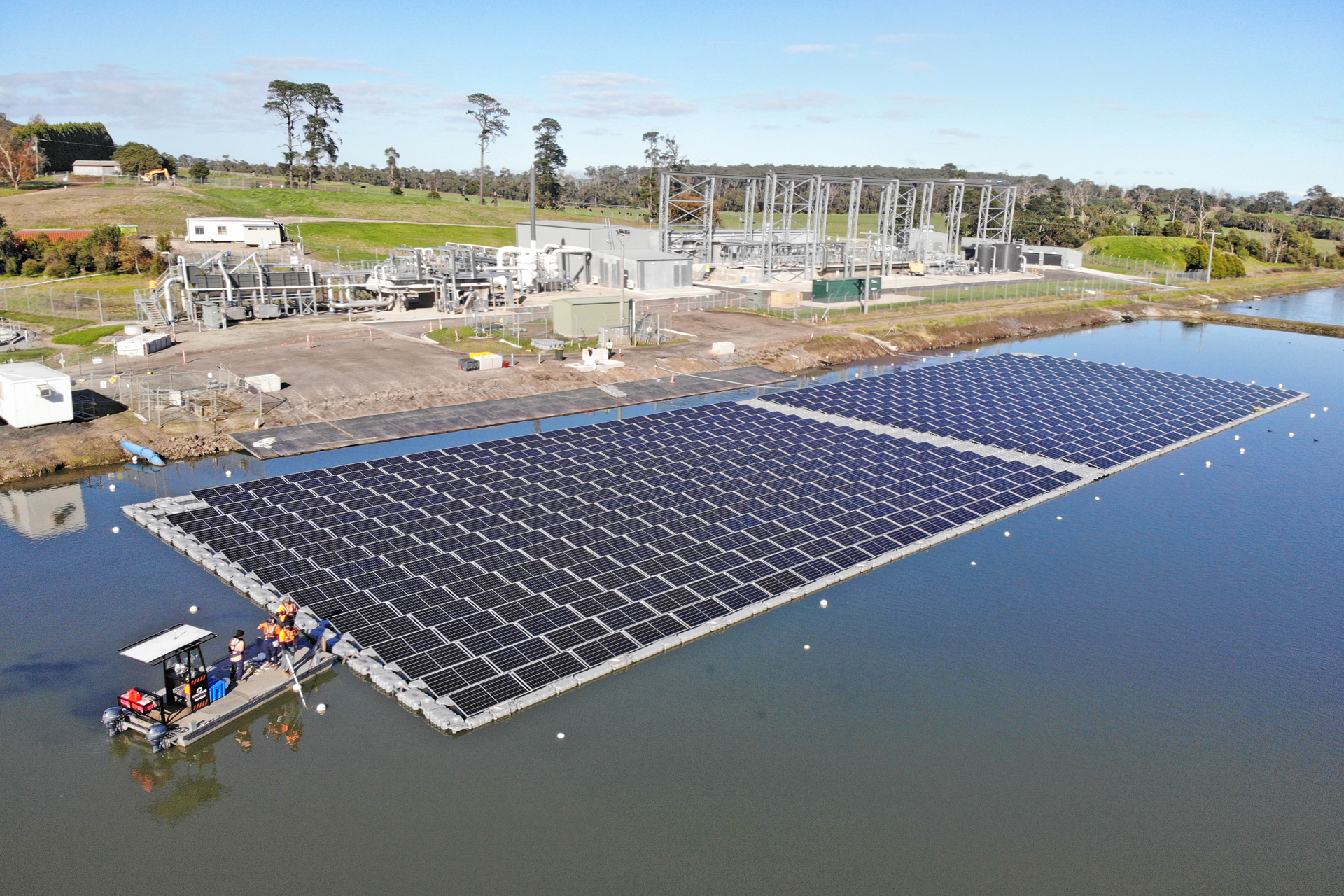Commercial
October 26, 2023

#### Greenwoood nominated for CEC solar awards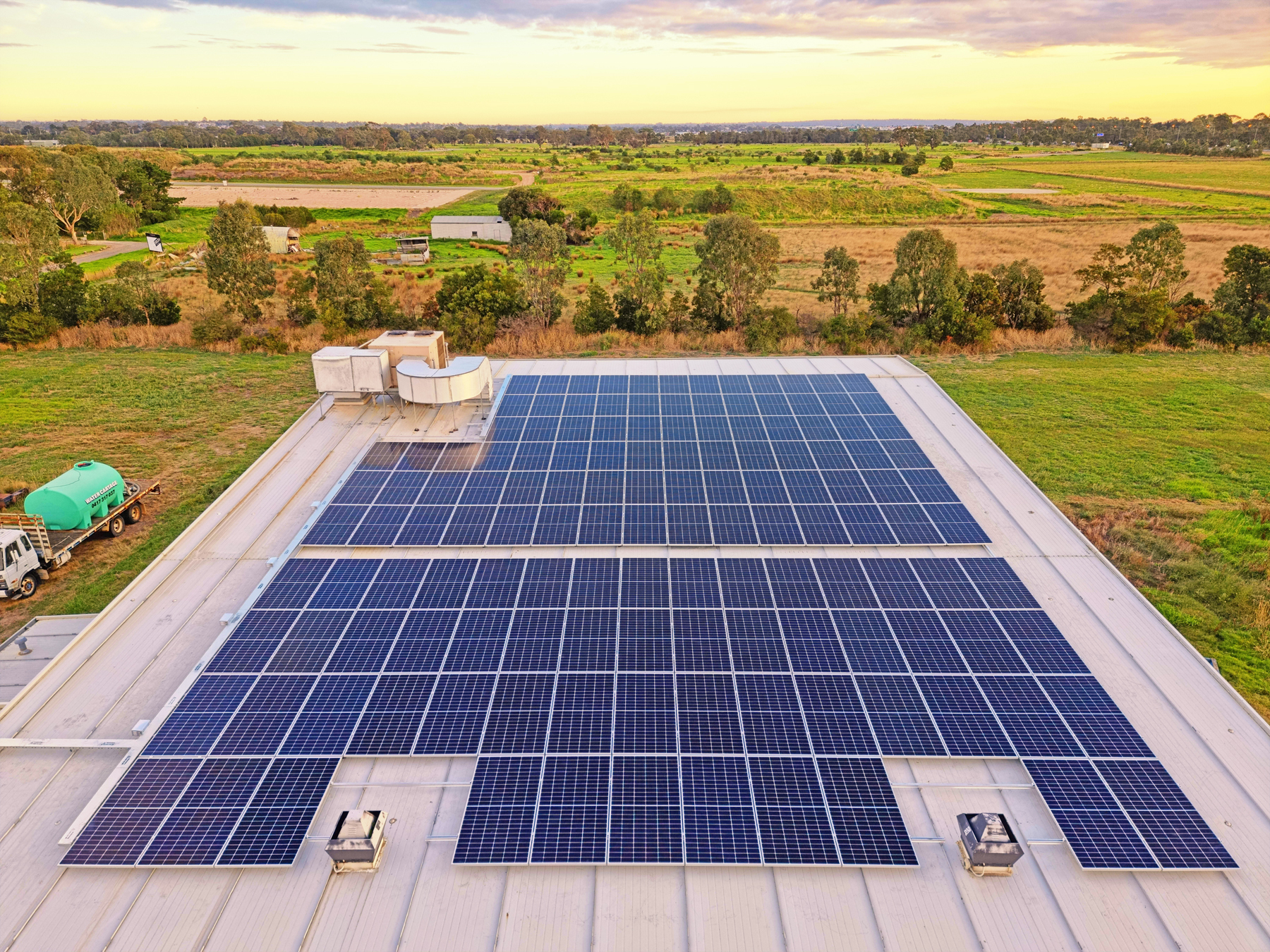Commercial
September 6, 2022

#### Commercial solar for a fish farm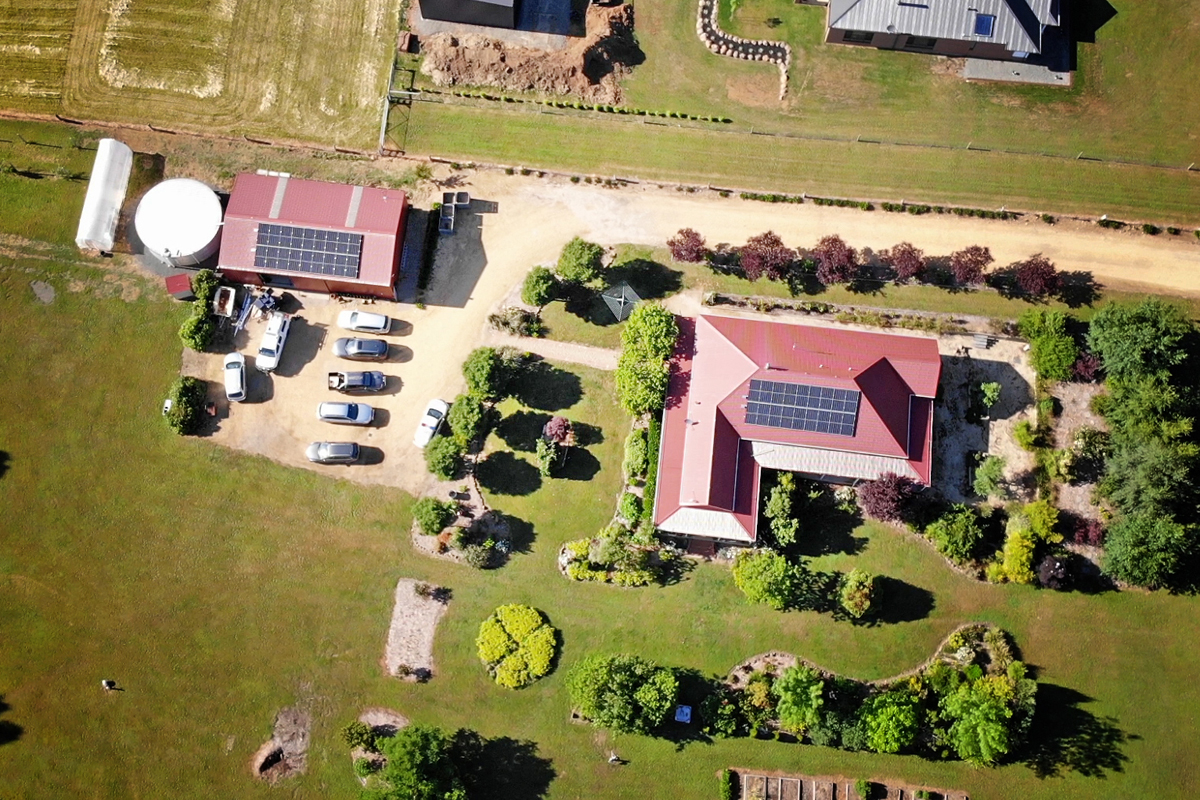Commercial
August 9, 2022

#### Trentham solar & battery: revisited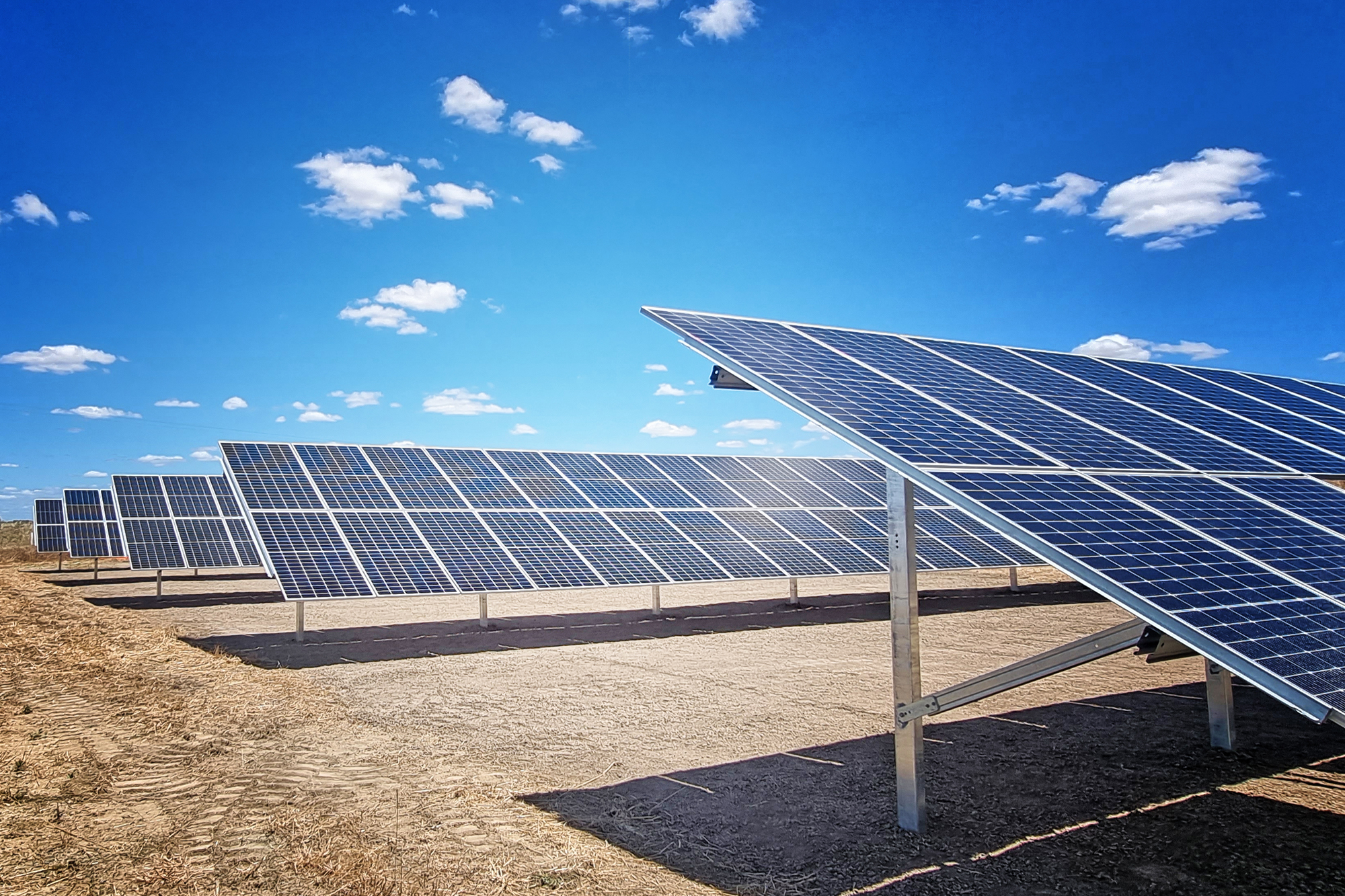Commercial
July 26, 2022

#### Ground Mount and AgrivoltaicsCommercial
July 20, 2022

#### Solar and batteries; life in the Mountains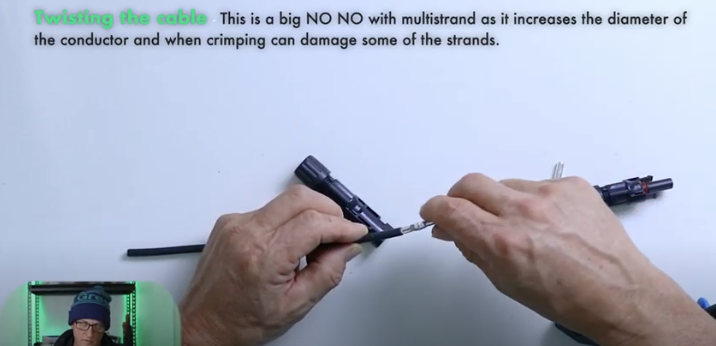Training
June 1, 2022

#### MC4 connections: how to crimp correctly